## research papersJOURNAL OFSYNCHROTRONRADIATION
ISSN: 1600-5775
Volume 13| Part 4| July 2006| Pages 343-345

## Sharp-focusing Bragg–Fresnel zone plate with Laue diffraction geometry

aYerevan State University, Alex Manoogian St. 1, Yerevan 375025, Armenia
*Correspondence e-mail: levhar@web.am

(Received 31 October 2005; accepted 18 May 2006)

The impact of decreased zone height on the focal properties of hard X-ray Bragg–Fresnel zone plates has been studied by numerical simulation. Decreased zone height allows for smaller zone widths and, although the efficiency of the lens is decreased, the signal-to-background ratio in the focal plane of the lens remains at a comparatively high level. This is distinct from an analogous case of ordinary phase zone plates.

### 1. Introduction

For years, zone-plate optics have achieved the sharpest focused X-ray beams in the soft X-ray regime and have continued to develop for hard X-ray applications where they similarly have achieved the narrowest beams (Chao et al., 2005; Suzuki et al., 2005). Recent emphasis on nanophase materials makes it essential to produce very narrow beams, and there is a global race to produce ever sharper focused hard X-ray beams for the study of nanoscale materials. The use of ordinary refractive lenses for focusing hard X-rays is complicated due to the extremely small difference in the refractive index of matter from unity (1 − n ≃ 10−5–10−6). As a consequence, alternative methods of focusing are used, one of which is the use of Fresnel zone plates. Both ordinary Fresnel zone plates (FZPs) and Bragg–Fresnel zone plates (BFZPs) are used. In the second case, Bragg diffraction is combined with spatial modulation of reflected waves according to the law of Fresnel zones. The latter is achieved if an appropriate relief is patterned on the exit surface of the zone plate; the phase of the diffracted wave is modulated by the Fresnel zones of different thicknesses. Two cases of BFZPs are distinguished: (i) the Bragg case, where the reflected wave leaves the same surface on which the primary wave is incident; and (ii) the Laue case, where the reflected wave leaves the opposite surface (Fig. 1). An important constraint on BFZP optics is the rise of edge effects in X-ray diffraction on lateral surfaces of zone structure that essentially distort the expected spatial modulation of the reflected radiation.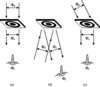Figure 1 Focusing of X-rays by means of (a) FZP, BFZP in (b) Bragg and (c) Laue diffraction geometries. Φf is the energy flux in the focal peak, Φ0 is the energy flux of radiation incident on the lens, and Φe is the energy flux of the wave package leaving the lens that falls on the focal plane after propagation in a vacuum.

In the Bragg diffraction case the problem is solved by using diffraction geometry close to backscattering, where the Bragg angle differs from 90° by only several degrees (Basov et al., 1991; Snigirev, 1995). From an experimental point of view, there are definite inconveniences attached to this geometry.

The Laue diffraction case was considered by Haroutunyan et al. (2005). It was shown by numerical simulation of dynamical diffraction of X-rays on BFZPs that the negative influence of edge effects may be suppressed by using a diffraction geometry where the wavevector of the reflected radiation is perpendicular to the surface of the zone plate. The optimal values of the basic parameters of BFZPs were found. It has been shown that in this case an amplitude–phase modulation of the reflected wave took place and edge effects even improve the focusing conditions by increasing the depth of the amplitude modulation and the total intensity of the reflected radiation. The efficiency of this lens exceeds the theoretical efficiency limit for an amplitude-modulated FZP.

In the present work the feasibility of modifying the parameters of the above-mentioned BFZP (with Laue diffraction geometry), to further increase the sharpness of focusing, is considered.

### 2. Sharp-focusing Bragg–Fresnel lens

Efficiency considerations for BFZPs differ from FZPs. As a consequence, along with the conventional efficiency coefficients (η = Φf/Φ0), a new parameter, ηf = Φf/Φe, is introduced as a supplementary characteristic of Fresnel lenses. Here, Φf is the energy flux in the focal peak, Φ0 is the energy flux of radiation falling on the lens, and Φe is the energy flux of a wave package leaving the lens which, after propagation in a vacuum, is incident on the focal plane (Fig. 1). If η characterizes the lens in the sense of usage efficiency of the energy of incident radiation, ηf characterizes the quality of the formed image in the sense of signal-to-background ratio. The condition ΦeΦ0 is satisfied for conventional optical refraction lenses as well as for phase-modulating FZPs, and, hence, ηfη (neglecting, of course, the absorption in the lens). For amplitude-modulating FZPs we have ΦeΦ0/2 and, hence, ηf ≃ 2η = 20%. In the case of BFZPs, ΦerΦ0 and, hence, ηfη/r, where r is an average reflection coefficient of the zone plate. As usual, the reflection coefficient r in the Bragg case is close to unity, while in the Laue case it is considerably less (Authier, 2001).

It is known that the half-width of the focal peak (Λf) of Fresnel zone plates is determined ultimately by the widths of the outermost (narrowest) zones (Λmin). With the present zone-plate fabrication technology the primary restriction is not as much on the value of Λmin as on the ratio Λmin/h, where h is the height of the Fresnel zones. Thus, the problem of increasing the sharpness of focusing is reduced to decreasing Λmin, which in turn requires a decrease of h.

The optimal value of h for FZPs (in the following the abbreviation FZP will mean the phase-modulated FZP) is determined by the equality of π to the phase difference of beams passing through the even and odd zones, h0 = λ/2(1 − n), where n is the refraction index of the zone plate and λ is the wavelength of the focused radiation. The depth of the phase modulation decreases with further decreasing h from h0. As a result, Φf decreases with almost constant Φe. In consequence, η → 0 and ηf → 0, when h → 0.

The situation for BFZPs with Laue diffraction geometry is somewhat different. This distinction is made apparent by the example of BFZPs in the absence of a substrate. Consider the geometry in which the wavevector of the reflected radiation is perpendicular to the zone-plate surface (Fig. 2a). As may be seen in the figure, the reflected wavefield in the geometrical shadow of the zone plate is amplitude-modulated, with zero intensity at its minima. The depth of modulation does not depend on h, although the mean beam intensity tends to zero when h → 0. Hence, when h → 0 we have Φe → 0, η → 0 and ηf ≃ 20%. Thus, in this scheme, a reduction of h will increase the sharpness of the focusing while keeping the parameter ηf at an acceptable level, in contrast to the case of standard FZPs. Nevertheless, this scheme is only illustrative in itself and is hardly suitable for practical applications because of the extremely low efficiency [for λ = 1 Å radiation, Si(220) reflection and h = 6 µm, η = 4.7%], as well as the difficulty of fabricating a zone plate without a substrate.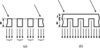Figure 2 Diffraction of X-rays in BFZPs (Laue reflection geometry) in (a) the absence and (b) the presence of a substrate (the portion of cross section with the scattering plane). t is the thickness of the zone-plate substrate and h is the height of the Fresnel zones.

The presence of a thin substrate (Fig. 2b) may improve the situation. Although in this case the depth of the amplitude modulation is reduced, the intensity of the reflected radiation sharply increases.1 In addition, a phase modulation arises that promotes focusing. Calculations of focusing parameters have been carried out based on a numerical simulation of dynamical diffraction of X-rays in BFZPs2 (Haroutunyan et al., 2002) (analytical calculations are complicated because of the complex boundary conditions imposed on the wave equation at lateral surfaces of the zone structure). The number of Fresnel zones (n) was chosen to be a function of h and be determined by the condition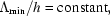where Λmin = Λ0[n1/2 − (n − 1)1/2], with Λ0 the radius of the first Fresnel zone. As discussed above, h proved to be the main factor defining the value of Λf and must be selected based on the requirements to the sharpness of focusing.

The calculations provide evidence of a complicated dependence of the focusing characteristics on the substrate thickness (t) and the displacement of incident radiation from the exact Bragg direction (Δθ0). This is connected in part with edge effects arising in X-ray diffraction on lateral surfaces of the zone structure. The optimal values of parameters t and Δθ0 are chosen by means of minimizing the function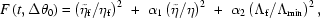reflecting the compromise between the basic focusing characteristics ηf, η and Λf. Here,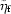= 0.2,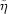= 0.1 (which are the values of parameters ηf and η, respectively, for the amplitude zone plates), and α1 and α2 are weighting coefficients defining the above compromise. The results of calculations for different values of h [λ = 1 Å radiation, Si(220) reflection, focal distance 0.5 m, α1 = 0.5, α2 = 1] are given in Table 1. For comparison, we also give here the results of calculations for analogous FZPs with the same values of h and h = h0. As expected, the sharpness of focusing for optimized BFZPs increases with decreasing h. Although in this case the efficiency of the lens falls, the parameter ηf remains at a comparatively high level. In particular, in the case of h = 6 µm, the focusing sharpness of optimized BFZPs is more than three times as high as that for FZPs with h = h0 (Λf = 0.2 µm instead of 0.61 µm). In this case, although the parameters η and ηf are considerably reduced (η = 11.1% and ηf = 24.6% instead of 32.7% and 34.5%, respectively), they nevertheless exceed corresponding values for the amplitude zone plates. Although in the case of FZPs with h = 6 µm the values of parameters Λmin and η are close to those for the aforementioned BFZPs, ηf is, however, almost half as large (ηf = 12.8% instead of 24.3%).

 Table 1Basic parameters of optimized BFZPs and analogous FZPs for different values of h, and FZPs with h = h0 (last row)
BFZP optimization variables Focusing parameters
h (µm) Λmin (µm) Lens type t (µm) Δθ0 (″) η (%) ηf (%) Λf (µm)
3.0 0.12 BFZP 5.4 2.5 3.4 11.3 0.10
FZP   3.5 3.6 0.10
4.0 0.16 BFZP 5.5 2.3 5.7 16.6 0.13
FZP   6.0 6.2 0.13
5.0 0.2 BFZP 6.1 2.0 8.4 20.8 0.17
FZP   9.0 9.4 0.18
6.0 0.24 BFZP 6.4 1.8 11.1 24.6 0.20
FZP   12.3 12.8 0.22
7.0 0.28 BFZP 6.8 1.6 13.8 27.3 0.24
FZP   15.8 16.5 0.25
8.0 0.32 BFZP 7.3 1.4 16.3 29.1 0.27
FZP   19.2 20.1 0.29
9.0 0.36 BFZP 7.3 1.3 18.0 30.6 0.30
FZP   22.6 23.7 0.33
15.8 0.64 FZP 5.0 32.7 34.5 0.61

In Fig. 3the calculated intensity distributions are plotted in the vicinity of the focal peak for the optimized BFZPs with h = 6 µm (a), FZPs with h = 6 µm (b), and FZPs with h = h0 (c). As may be seen from these plots, in the case of optimized BFZPs we have both small peak half-width and low background for the loss of intensity.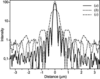Figure 3 Intensity profiles for (a) the optimized BFZPs with h = 6 µm, (b) FZPs with h = 6 µm and (c) FZPs with h = h0. In case (c) the high level of background is related to the small radius of the lens. The intensity of radiation incident on the lens is taken as the intensity unit.

The fact that the number of zones and hence the radius of the BFZP increases with the reduction of h, and accordingly Λmin, is also important. For a sufficient incident-beam width, this may partially compensate for the fast falling of the lens efficiency.

### 3. Conclusion

The possibility of modifying BFZPs in the Laue diffraction geometry, causing an increase in the sharpness of focusing due to the drop in lens efficiency, is shown. This modification is achieved because of a decrease in the height and, accordingly, the minimal width of the Fresnel zones. Although in this case the lens efficiency is reduced, the parameter ηf, describing the signal-to-background ratio in the focal plane of the lens, remains at a comparatively high level, unlike the similar FZP case. The mentioned drop of lens efficiency is partly compensated for by an increase in zone-plate radius, caused by a reduction of the minimal width of the Fresnel zones.

The optimal value of the height of Fresnel zones is determined by a compromise between the sharpness and lens efficiency, and must be selected based on specific experimental conditions.

### Footnotes

1It is known that the intensity of the reflected radiation is proportional to the square of the thicknesses of the crystalline plate, if the latter is much smaller than the extinction distance.

2For the purpose of the decreasing CPU time, the calculations indicated are carried out for the one-dimensional zone plates, when zones are directed perpendicularly to the scattering plane.

### ReferencesJOURNAL OFSYNCHROTRONRADIATION